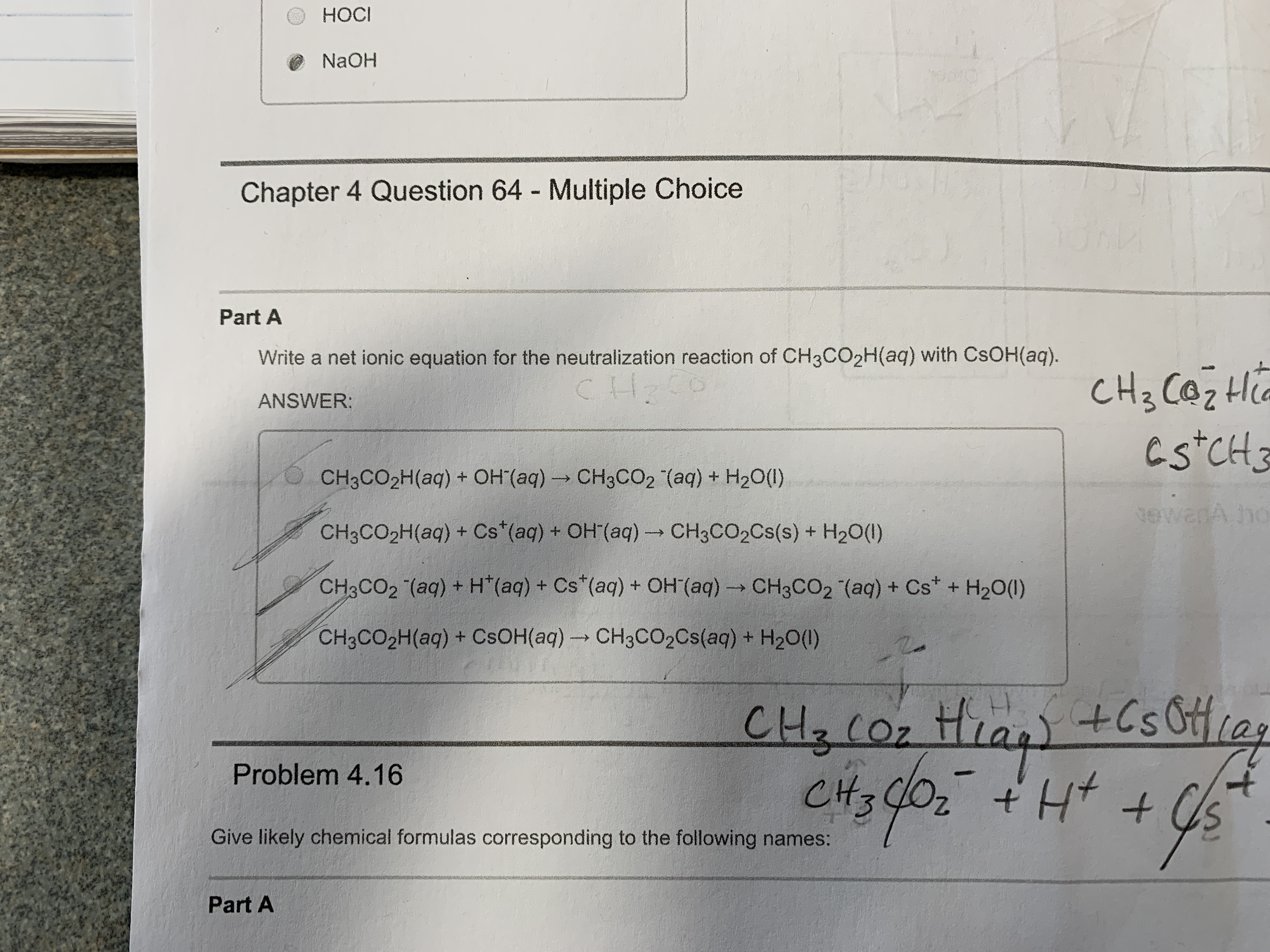# HOCI НОСI NAOH Chapter 4 Question 64 - Multiple Choice Part A Write a net ionic equation for the neutralization reaction of CH3CO2H(aq) with CSOH(aq) CL CH3 CazH Cs CH ANSWER: CH3CO2H(aq) + OH (aq) CH3CO2 (aq) + H20() mache tewanA ho CH3CO2H(aq) + Cs (aq) + OH(aq)- CH3CO2CS(s) + H20(1) CH3CO2 (aq) + H (aq) + Cs (aq) + OH (aq) CH3CO2 (aq) + Cs+ H20() CH3CO2H(aq) + CSOH(aq) CH3CCO2CS(aq) + H20() +CsOias CH Coz t CH3 Problem 4.16 Give likely chemical formulas corresponding to the following names: AHp Part A

Question

Write the net ionic equation for the neutralization reactionhelp_outlineImage TranscriptioncloseHOCI НОСI NAOH Chapter 4 Question 64 - Multiple Choice Part A Write a net ionic equation for the neutralization reaction of CH3CO2H(aq) with CSOH(aq) CL CH3 CazH Cs CH ANSWER: CH3CO2H(aq) + OH (aq) CH3CO2 (aq) + H20() mache tewanA ho CH3CO2H(aq) + Cs (aq) + OH(aq)- CH3CO2CS(s) + H20(1) CH3CO2 (aq) + H (aq) + Cs (aq) + OH (aq) CH3CO2 (aq) + Cs+ H20() CH3CO2H(aq) + CSOH(aq) CH3CCO2CS(aq) + H20() +CsOias CH Coz t CH3 Problem 4.16 Give likely chemical formulas corresponding to the following names: AHp Part A fullscreen

### Want to see this answer and more?

Experts are waiting 24/7 to provide step-by-step solutions in as fast as 30 minutes!*